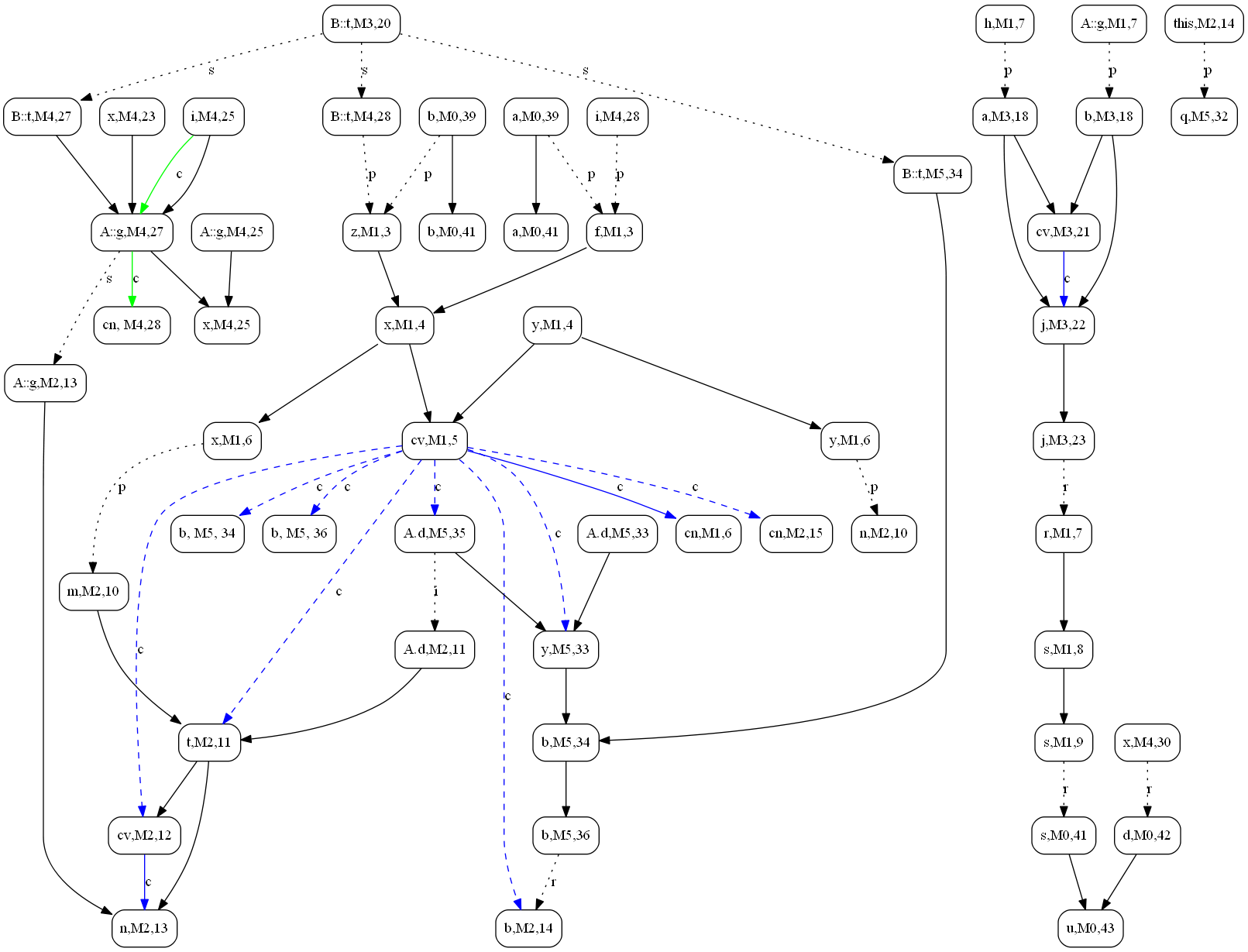# Illustration of the MDG

## How Diver Works

The core technique of Diver is the static dependence modeling via the graph representation model---the MDG---that models all dependencies at the finest statement level.

We illustrate how the MDG looks like by presenting on this page an example Java program and its static MDG created by Diver.

### Example Program

```
/* example code for Diver */
public class A {
static int g; public int d;
String M1(int f, int z) {
int x = f + z, y = 2, h=21;
if (x > y) {
M2(x,y);
}
int r = new B().M3(h,g);
String s = "M3 retval: " + r;
return s;
}

void M2(int m, int n) {
int t = m - d;
if (t > 0) {
n = g/t;
}
boolean b = C.M5(this);
//System.out.println(b);
}
}

/* example code for Diver */
public class B {
static short t;

int M3(int a, int b) {
int j = 0;
t = -250;

if ( a < b ) {
j = b - a;
}
return j;
}

static double M4() {
int x = A.g, i = 5;

try {
A.g = x/(i+t);
new A().M1(i, t);
}
catch(Exception e) {	}

return x;
}
}

/* example code for Diver --- this is the entry class */
public class C {
}

public static boolean M5(A q) {
long y = q.d;
boolean b = B.t > y;
q.d = 2;
return b;
}

public static void main(String[] args) {
int a = 0, b = -3;
String s = new A().M1(a,b);
double d = B.M4();
String u = s + d;
System.out.println(u);
}
}

```

### Static MDG of the Example Program

Meanings of labels and color markings in the example MDG diagram are as follows:

• p -- parameter edge
• r -- return edge
• i -- instance field edge
• s -- static field edge
• c -- control dependence edge
• solid back line -- intraprocedural edges
• dotted gray line -- interprocedural data dependence edge
• dashed blue line -- interprocedural control dependence edge
• solid blue line -- intraprocedural control dependence edge
• solid green line -- exceptional control dependence edgeNote:
This diagram is a conceptual illustration only;
The real MDG that Diver created and used for impact analysis is based on the Soot Jimple IR (an immediate representation of Java code transformed from the Java byte-code).

Generated on 14 Mar 2014 by1.6.1